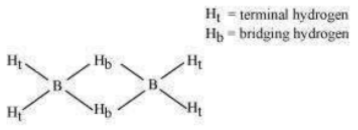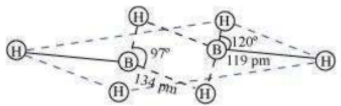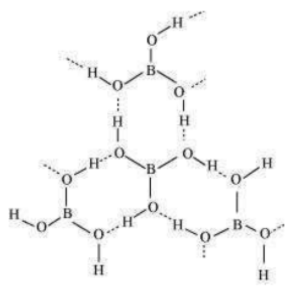Explain structures of diborane and boric acid.

(a) Diborane
${B}_{2}{H}_{6}$ is an electron-deficient compound. ${B}_{2}{H}_{6}$ has only 12 electrons – 6 ${e}^{-}$ from 6 H atoms and 3 ${e}^{-}$ each from 2 B atoms. Thus, after combining with 3 H atoms, none of the boron atoms has any electrons left. X-ray diffraction studies have shown the structure of diborane as:2 boron and 4 terminal hydrogen atoms (${H}_{t}$) lie in one plane, while the other two bridging hydrogen atoms (${H}_{b}$) lie in a plane perpendicular to the plane of boron atoms. Again, of the two bridging hydrogen atoms, one H atom lies above the plane and the other lies below the plane. The terminal bonds are regular two-centre two-electron (2c – 2${e}^{-}$ ) bonds, while the two bridging (B–H–B) bonds are three-centre two-electron (3c – 2${e}^{-}$ ) bonds.(b) Boric acid
Boric acid has a layered structure. Each planar $B{O}_{3}$ unit is linked to one another through H atoms. The H atoms form a covalent bond with a $B{O}_{3}$ unit, while a hydrogen bond is formed with another $B{O}_{3}$ unit. In the given figure, the dotted lines represent hydrogen bonds.